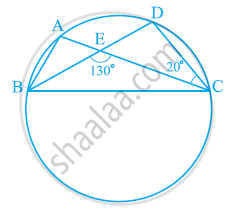# In the given figure, A, B, C and D are four points on a circle. AC and BD intersect at a point E such that ∠BEC = 130° and ∠ECD = 20°. Find ∠BAC - Mathematics

In the given figure, A, B, C and D are four points on a circle. AC and BD intersect at a point E such that ∠BEC = 130° and ∠ECD = 20°. Find ∠BAC.#### Solution

In ΔCDE,

∠CDE + ∠DCE = ∠CEB (Exterior angle)

⇒ ∠CDE + 20° = 130°

⇒ ∠CDE = 110°

However, ∠BAC = ∠CDE (Angles in the same segment of a circle)

⇒ ∠BAC = 110°

Is there an error in this question or solution?

#### APPEARS IN

NCERT Class 9 Maths
Chapter 10 Circles
Exercise 10.5 | Q 5 | Page 185